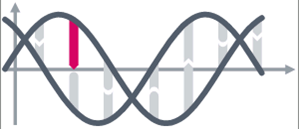### Mathematics in Oncology

Cancer is one of the leading causes of disease-related death worldwide. In recent years, rapid increase in the molecular understanding of cancer has unraveled significant additional complexity of the disease. Although large amounts of data on cancer genetics and molecular characteristics are available and accumulating with increasing speed, adequate interpretation of these data still represents a major bottleneck. This is exactly where mathematics can be applied to oncology: Through mathematical modeling of complex biological processes we are able to gain novel, unprecedented medical insights. The fields of application of mathematical models include the analysis of biological concepts and medical hypotheses about cancer evolution, and the prediction of clinical outcomes using existing clinical and molecular information. On the other hand, the medical applications give rise to mathematical challenges, which can lead to new methods and algorithms in various fields of mathematics, like data analysis, mathematical modeling and machine learning. Therefore, applying mathematics in the field of oncology will facilitate data interpretation and improve our understanding of carcinogenic processes.

https://emcl.iwr.uni-heidelberg.de/research/projects/mathematical-oncology

#### PROJECT COLLABORATORS

Engineering Mathematics and Computing Lab (EMCL)

Prof. Dr. Vincent Heuveline, IWR, Heidelberg University